# Investigation of thermal conductivity for liquid metal composites using the micromechanics-based mean-field homogenization theory†

Jiyoung Junga, Seung Hee Jeong*b, Klas Hjortb and Seunghwa Ryu*a
aDepartment of Mechanical Engineering, Korea Advanced Institute of Science and Technology (KAIST), 291 Daehak-ro, Yuseong-gu, Daejeon 34141, Republic of Korea. E-mail: ryush@kaist.ac.kr
bDivision of Microsystems Technology, Department of Engineering Sciences, The Angstrom Laboratory, Uppsala University, Box 534, SE 751 21, Uppsala, Sweden. E-mail: seunghee.jeong@angstrom.uu.se

Received 17th February 2020 , Accepted 2nd May 2020

First published on 6th May 2020

For the facile use of liquid metal composites (LMCs) for soft, stretchable and thermal systems, it is crucial to understand and predict the thermal conductivity of the composites as a function of liquid metal (LM) volume fraction and applied strain. In this study, we investigated the effective thermal conductivity of LMCs based on various mean-field homogenization frameworks including Eshelby, Mori–Tanaka, differential and double inclusion methods. The double inclusion model turned out to make the prediction closest to the experimental results in a wide range of LM volume fractions. Interestingly, we found that the theoretical models based on the assumption of ideal LM dispersion and zero interfacial resistance underestimated the thermal conductivity compared to the experimental results in a low volume fraction regime. By considering the accompanied variations in the LM inclusion's aspect ratios under a typical size distribution of inclusions (∼μm), the change of effective thermal conductivity was predicted under a uniaxial 300% tensile strain. Our study will deepen the understanding of the thermal properties of LMCs and support the designs of stretchable thermal interfaces and packaging with LMCs in the future.

## Introduction

Soft and stretchable materials are promising for the development of stretchable electronics, soft robotics and wearable systems.1–3 Various soft and stretchable sensors and actuators have been demonstrated with silicone-based materials, but soft and stretchable thermal management materials that are compatible with the aforementioned applications are still under development. Liquid metal composites (LMCs), which are liquid composites composed of a silicone elastomer matrix and liquid metal (LM) droplets as inclusions, have great potential for soft and stretchable systems.4,5 LMCs are promising candidates for thermal interfaces and packaging that can form a compliant and stretchable thermal interface with electrical insulation. More importantly, the developed LMCs are moldable and curable as well as conformal on uneven surfaces. At the highest LM volume fraction, the thermal conductivity of LMCs is twelve times higher than that of a pure elastomer matrix.6

For the facile use of LMCs, it is important to understand and predict their thermal conductivity as a function of the LM volume fraction and applied strain, which would allow one to design LMCs for a specific application. One can employ numerical tools, such as finite element analysis, but multiple calculations are required to obtain statistically meaningful data by varying the positions and the number of inclusions within the representative volume elements (RVEs). As an alternative, theoretical models with cost-efficient analysis have been proposed for predicting the effective properties of composites. Mean field schemes such as the Maxwell–Garnett's model7 and Bruggeman's theory,8–10 differential schemes such as the Tavangar's model,11,12 and fast Fourier transform schemes13,14 have been applied to predict the effective thermal conductivity of composites such as LMCs. In this paper, we have considered the mean-field homogenization scheme based on the micromechanics theory and Eshelby's solution because of its convenience of handling a composite involving multiple types of inclusions (here, liquid inclusions have different sizes and different aspect ratios). Because of the mathematical analogy between the governing equations of elasticity and thermal conductivity, the Eshelby's theory on micromechanics has been adapted and applied to study the effective thermal properties.15,16

In this work, we have employed four different homogenization frameworks, namely, Eshelby,17,18 Mori–Tanaka,15,16,19 differential20–22 and double inclusion methods20,23 to predict the thermal conductivity of the LMC as a function of the LM volume fraction and an applied uniaxial strain. These methods are classified according to how the interaction between inclusions is (partially) incorporated. In the first half, we show that the double inclusion method matches the experimental results with the smallest error in a wide range of LM volume fraction and discuss why it is so. We then investigated the unusual discrepancy between the mean-field theoretical prediction and experiments, namely, the underestimation of thermal conductivity even in the idealized LM droplet dispersion and zero interfacial thermal resistance assumptions. We hypothesize the origin of the discrepancy in terms of the LM aspect ratio as a candidate cause of the discrepancy and a few plausible explanations on the discrepancy are discussed. In the second half of the paper, we predict the change of the thermal conductivity of LMC under tension, by predicting the aspect ratio change of LM inclusions. Interestingly, the thermal conductivity of LMC is shown to change by almost a factor of three. We conclude the paper by discussing the implication and future direction of the present study.

## Theoretical models

### Mean-field homogenization frameworks

In this section, we aim to predict the effective thermal conductivity of LMC. The governing equation and constitutive equation in a steady state heat conduction are as follows, with boldfaced letters representing matrices and vectors,
 ∇·q(x) − g(x) = 0, (1)
 q(x) = Ke(x) where e(x) = −∇T(x), (2)
where q is the heat flux, g is the heat generation per unit volume, K is the thermal conductivity tensor, e is the intensity field and T is the temperature. Here, K is a 3 × 3 symmetric second order tensor, q and e are three-dimensional vectors and T and g are scalar fields. A binary composite consists of the matrix phase and an inhomogeneity phase that has different physical properties from the matrix. By applying an appropriate fictitious eigen-intensity field e* in the well-known Eshelby's solution for a single inhomogeneity in the infinite matrix, the inhomogeneity is equivalently replaced with an inclusion that has identical physical properties with the matrix, as shown in Fig. 1(a). The eigen-intensity field e* satisfies the following equation, so that the heat flux within the inhomogeneity is identical to the heat flux within the inclusion,
 K1(e0 + ept) = K0(e0 + ept − e*), (3)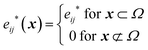(4)
where K1 is the thermal conductivity tensor of the inhomogeneity, K0 is the thermal conductivity tensor of the matrix, e0 is the exterior intensity field by exterior heat flux q0 (q0K0e0), and ept is a perturbation term due to the presence of an inhomogeneity. The indices on subscripts run from 1 to 3 which represent the three components of the Cartesian coordinate system. The left side of the eqn (3) represents the heat flux within the inhomogeneity and the right side of the eqn (3) represents the heat flux within the equivalent inclusion. The Eshelby tensor S, which maps the eigen-intensity field to the perturbation intensity field, is defined as,
 ept ≡ Se*. (5)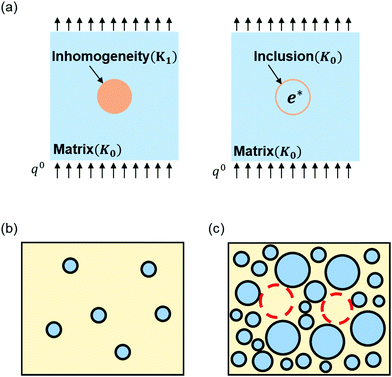Fig. 1 (a) Schematic configurations of inhomogeneity and an equivalently replaced inclusion in a matrix. The inhomogeneity in a matrix has different material properties from the matrix, while the inclusion has identical material properties with the matrix. The fictitious eigen-intensity field e* is applied over the inclusion domain. (b and c) Schematic configuration of LMC of (b) sparsely distributed inclusions at a low volume fraction and (c) densely distributed inclusions at a high-volume fraction. Red dashed lines show the parts where the matrix phase is considered as inclusions surrounded by liquid inclusions for the double inclusion method.

Obtaining the proper Eshelby tensor is the most important in applying the homogenization framework based on the Eshelby's solution. The Eshelby tensor is a function of material properties of a matrix and the shape of an inclusion. In heat conduction problems, the Eshelby tensor with an anisotropic matrix and ellipsoidal inclusion is derived as follows,16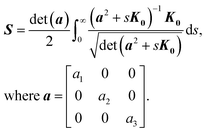(6)
Here, a1, a2, and a3 are the half-length of the principal axes along the x, y, and z directions of the ellipsoidal inclusion, respectively. When the matrix has isotropic thermal conductivity and the inclusion has a prolate spheroid shape (a1 > a2 = a3) or oblate spheroid (a1 < a2 = a3) with x-axis symmetry, the Eshelby tensor is reduced as follows,24
 S11 = 1 − 2S22, (7)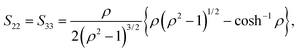(8)
where the aspect ratio ρ is defined as a1/a2. For an isotropic matrix with a spherical inclusion, the Eshelby tensor is reduced as follows,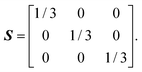(9)

When a single inclusion exists in the infinite matrix, substituting eqn (5) into (3), and the local concentration tensor T, which maps the exterior intensity field to the intensity field within the inclusion e1, can be obtained as follows,

 T = [I + SK0−1(K1 − K0)]−1, (10)
where
 e1 = e0 + ept = Te0 (11)
and I is the 3 × 3 identity matrix.

By extending Eshelby's solution on a single inhomogeneity to multiple inhomogeneity problems with the appropriate approximations, various homogenization techniques have been developed, such as the Eshelby method, Mori–Tanaka method, the differential method, the double inclusion method and others. Considering the volume average over composites, the volume averaged heat flux and intensity field satisfy the following equations,

 〈q〉 = c0〈q0〉 + c1〈q1〉 = c0K0〈e0〉 + c1K1〈e1〉, (12)
 〈q〉 = Keff〈e〉, (13)
where 〈·〉 refers to the volume average of the physical value, and c0 and c1 are the volume fractions of the matrix and inclusion, respectively. Subscript 0 and 1 refer to the physical quantity of matrix and inclusion, respectively. Using eqn (12) and (13), the effective thermal conductivity tensor Keff of LMC is reduced as follows,
 Keff = K0 + c1(K1 − K0)A where 〈e1〉 = A〈e〉, (14)
where A is the global concentration tensor. The Eshelby method assumes that each inclusion is an isolated inclusion that does not interact with other inclusions. Hence, the interaction between inclusions is neglected and the global concentration tensor is assumed to be identical to the local concentration tensor (AT). The effective thermal conductivity tensor KEshelbyeff of an LMC derived by the Eshelby method is represented as follows,
 KEshelbyeff = K0 + c1(K1 − K0)T. (15)

Since the Eshelby method assumes an isolated inclusion, the theoretical prediction by the Eshelby method is known to be valid in a very dilute volume fraction of inclusion.

As an alternative to the Eshelby method, the Mori–Tanaka method reflects interactions between inclusions by assuming that an intensity field within a matrix around each inclusion is equal to the volume averaged intensity field of the matrix (e0(x) = 〈e0〉). The global concentration tensor and the effective thermal conductivity tensor in the Mori–Tanaka method are derived as follows,

 A ≈ T(c0I + c1T)−1, (16)
 KMTeff = K0 + c1(K1 − K0)T(c0I + c1T)−1 = (c0K0 + c1K1T)(c0I + c1T)−1. (17)

The Mori–Tanaka method is known to be valid for up to 20% of the volume fraction.

The differential method was developed to predict the effective properties of composites with moderate finite volume fractions. The differential method is proposed with the idea that the prediction from the Eshelby/Mori–Tanaka methods is reliable at low volume fractions. The differential scheme homogenization proceeds as follows. First, a small amount (Δc1) of inclusions is added to the matrix with K0 and homogenization is applied, resulting in a homogenized phase with K(1)eff. Second, a small amount (Δc1) of inclusions is added to the homogenized phase and homogenization is applied again. Third, the previous step is repeated until a desired volume fraction is reached and an effective thermal conductivity by the differential method KDiffeff is obtained. Here, a small enough incremental volume fraction Δc1 is chosen by testing the convergence over Δc1 reduction. Applying the differential method based on the Mori–Tanaka approach, the effective thermal conductivity tensor is derived as follows,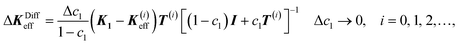(18)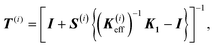(19)
where K(0)eff refers to the thermal conductivity tensor of the matrix K0, K(i)eff refers to the effective thermal conductivity tensor after the i-th homogenization, and S(i) refers to the Eshelby tensor derived using K(i)eff. The differential method is known to be valid at a moderate finite volume fraction.

The double inclusion method was developed to predict the effective properties of composites with arbitrary volume fractions. When inclusions with low volume fractions are sparsely distributed as shown in Fig. 1(b), the effective properties can be predicted by applying the Mori–Tanaka method. Similarly, with eqn (10), a local concentration tensor at a low volume fraction is derived as follows,

 Tlow = [I + SK0−1(K1 − K0)]−1. (20)

On the other hand, when inclusions with a very high-volume fraction are densely distributed as shown in Fig. 1(c), the matrix phase seems to be surrounded by inclusions. Considering the configuration of the matrix phase wrapped by inclusions, the local concentration tensor with a high-volume fraction is reduced as follows,

 Thigh = [I + SK1−1(K0 − K1)]. (21)

In the double inclusion scheme, the local concentration tensor TD is obtained by smoothly interpolating Tlow and Thigh’ using an appropriate interpolation function ζ. The interpolation function ζ should satisfy the following conditions,20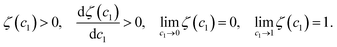(22)

We set the volume fraction of inclusions as an interpolation function, and the local concentration tensor in the double inclusion method is represented as follows,

 ζ(c1) = c1, (23)
 TD = (1 − ζ)Tlow + ζThigh. (24)

Then, the effective thermal conductivity tensor in the double inclusion method KDIeff is obtained as follows,

 KDIeff = (c0K0 + c1K1TD)(c0I + c1TD)−1. (25)

### Thermal conductivity of LMCs

We predict the effective thermal conductivity tensors of the LMC consisting of a PDMS matrix and LM inclusions using the Eshelby, Mori–Tanaka, differential, and double inclusion methods, and compare the results with previous experimental results.6 The thermal conductivity of the LM (Galinstan) is 16.5 W m K and that of the PDMS (Elastosil RT601) is 0.17 W m K.25,26 Here, we use eqn (9) for the Eshelby tensor to consider the spherical LM inclusion embedded in the isotropic PDMS matrix. The presence of a gallium oxide layer on the surface of the LM droplet is neglected since the oxide layer is very thin and the difference of thermal conductivity between the LM and the oxide layer is not so large (the thermal conductivity of single crystal β-gallium oxide is reported as 10.9–27 W m K).27 In Fig. 2, we compare the theoretical predictions with experimental data. The results are normalized by the thermal conductivity of PDMS, κPDMS. The errors from each homogenization method are summarized in Table 1. The Mori–Tanaka method shows identical predictions with Maxwell–Garnett's model in the case of a spherical inclusion as noted by the previous studies28,29 (see the SI.1, ESI). Note that Bruggeman's model shows similar results with the double inclusion model with the interpolation function ζ(c1) = c1 (see the SI.1, ESI). The differential method appears to fit well below 40% of the volume fraction. The double inclusion method fits well overall in most of the volume fraction range.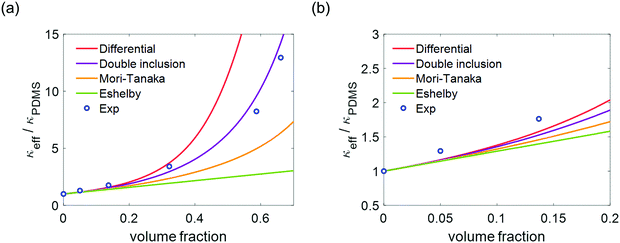Fig. 2 Different homogenization models for effective thermal conductivities of LMCs. (a) Effective thermal conductivities compared to the experimental data.6 The thermal conductivities are normalized by the thermal conductivity of PDMS. (b) A detailed plot from 0 to 20% of volume fraction.
Table 1 Relative errors for each homogenization method compared to experimental data.6 Errors over 100% are not shown
LM volume fraction 5.0% 13.7% 32.2% 58.7% 66.1%
Eshelby 11.49% 20.74% 43.23% 67.12% 77.41%
Mori–Tanaka 10.91% 17.28% 30.76% 39.72% 50.88%
Differential 9.98% 10.54% 9.06%
Double inclusion 10.32% 13.39% 13.41% 16.19% 10.16%

Interestingly, we find that the theoretical models based on ideal LM dispersion and zero interfacial resistance assumptions underestimate the thermal conductivity compared to the experimental results in a low volume fraction regime, as shown in Fig. 2(b). A similar phenomenon was observed in the previous studies predicting the Young's modulus of LMC.30,31 One can predict a few plausible causes of the discrepancy, such as (i) the non-spherical shape of the inclusions, (ii) the change of inherent property of PDMS upon fabrication or measurement variation, (iii) the effect of a thin oxide layer or interfacial thermal resistance and (iv) the limitation of mean-field homogenization.

First, we can exclude the third hypothesis because an interfacial thermal resistance would lower the effective thermal conductivity prediction even more and the conductivity of a thin oxide layer is comparable with that of the LM droplet. It is obvious that the mean-field homogenization framework cannot account for the effect of the local agglomeration of inclusions, but the agglomeration effect would lower the thermal conductivity. Because the theoretical model assumes the ideal dispersion of inclusions, the underestimation from the mean-field homogenization theory cannot be explained this way.

Second, regarding to the first hypothesis, we tested the effect of realistic inclusion shapes that deviate from a perfect sphere. According to the CT scan measurements on LMCs, due to the influence of a solid oxide layer, the LM inclusions actually have an ellipsoidal shape with different aspect ratios.9 The average aspect ratio was considered as the reported 1.5.9 We predicted the effective thermal conductivities of the LMCs with randomly oriented ellipsoidal inclusions in order to test whether the experimental value can be explained by accounting for realistic inclusion shape distribution. Following the previous studies of the orientation averaged Mori–Tanaka method,32,33 we can predict the thermal conductivity of LMCs with randomly oriented LM droplets as follows,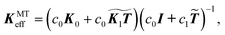(26)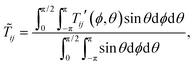(27)
 Tij′ = riprjqTpq, (28)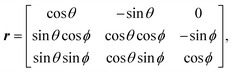(29)
where θ and ϕ are the azimuthal and polar angle, respectively, and r is a rotation matrix. Here, the local concentration tensor T is obtained using eqn (7), (8) and (10). We plotted the results obtained for the aspect ratios of 1 and 1.5, as shown in Fig. 3(a), and summarized the relative errors in Table 2 (see the SI.2, ESI). Although the discrepancy is reduced when an aspect ratio of 1.5 is used, the improvement turns out to be marginal (about 2% of the error reduction at 13.7% of the volume fraction).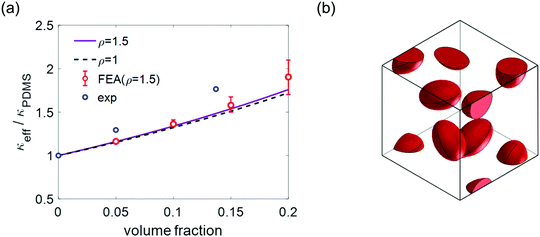Fig. 3 Inclusion shape effect on effective thermal conductivity estimation. (a) Normalized effective thermal conductivities of LMCs when aspect ratios are 1 and 1.5, respectively. Ellipsoidal inclusions are randomly oriented. (b) Configuration of randomly oriented ellipsoidal inclusion with the aspect ratio of 1.5 when volume fraction is 10%. Ten different specimens for each volume fraction are used for the Finite Element Analysis.
Table 2 Relative errors depending on the aspect ratios. Errors calculated by comparing theoretical prediction from an orientation averaged Mori-Tanaka method with experimental data6
LM volume fraction 5.0% 13.7%
ρ = 1 10.91% 17.28%
ρ = 1.5 10.29% 15.92%

To confirm our prediction, we conducted a finite element analysis (FEA) of the LMC involving randomly oriented ellipsoidal inclusions with an aspect ratio of 1.5 by generating ten different representative volume elements (RVEs) by Digimat-FE software, as shown in Fig. 3(b). The numerical results from the FEA show good agreement with the orientation averaged Mori–Tanaka method below 20% of the volume fraction, which implies that the theoretical framework is valid and also that the experimental data cannot be explained despite accounting for a realistic aspect ratio.

Finally, we tested the last possible cause of the discrepancy, which is the change of the inherent thermal conductivity of PDMS upon the fabrication of LMCs. We found that if the thermal conductivity of PDMS within LMCs increases by 10% (from 0.17 W m K to 0.187 W m K), the theoretical prediction matches better with the experimental data (see the SI.3, ESI). In that sense, we suspect that the thermal conductivity of PDMS might have changed after fabrication, or varied from the measurements, especially the PDMS conductivity on its own and in LMCs. This can be correlated with the measured thermal property variations of PDMS. This would also explain the observation in the previous studies, which show that the experimentally measured stiffness of LMC is higher than the mean-field homogenization theory prediction.30,31

### Thermal conductivity of LMCs subjected to uniaxial tension

Because one the most interesting applications of LMCs are soft and stretchable thermal interfaces and packaging, we studied the change of its thermal conductivity subjected to tensile deformation. Upon tensile loading of an LMC, the originally spherical inclusions deform into ellipsoidal shapes. The deformation of inclusions embedded in an LMC is resisted by the surface tension of inclusions, which depends on the elastocapillary length L.30,34 The elastocapillary length is defined as Lγ/E, where γ is the surface tension of a liquid inclusion and E is the Young's modulus of a solid matrix, which is PDMS in this case. The surface tension effect plays an important role if the size of inclusion is smaller than the elastocapillary length. The variation of the inclusion's axial length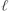can be analytically predicted for a single liquid droplet inclusion embedded in a matrix subjected to a far-field strain under a plane stress condition as follows,30,35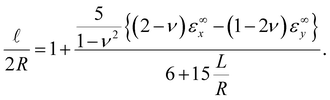(30)

Considering the volume conservation of an axisymmetric ellipsoidal inclusion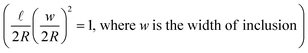, the aspect ratio ρ can be obtained as follows,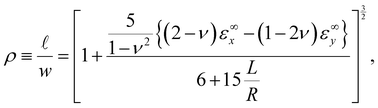(31)
where R is the radius of inclusion, ν is Poisson's ratio of a matrix and εx and εy are a far-field strain in the x and y-axis, respectively. Young's modulus and Poisson's ratio of PDMS are set to be 650 kPa and 0.5, respectively, and the surface tension of the LM droplet is set to be 718 mN m−1.36,37 The strain ratio of the lateral and transverse far-field strains is approximately assumed to be −0.2 (i.e. εy= −0.2εx, the strain ratio of a far-field strain could be different depending on the experimental environment), following the previous study.30 From this, one can obtain the aspect ratio as a function of the tensile strain εx and the radius of inclusion R.

We considered the typical LM inclusion size distribution, ranging from 2 μm to 50 nm of the diameters of inclusions, as reported in a previous study.6 We predict the effective thermal conductivity of the LMC with the 20% volume fraction, based on the double inclusion method because the double inclusion method showed the best match with experiments among four different methods in the previous section. Because we consider the relatively small volume fraction, the aspect ratio prediction for a single inclusion, eqn (30), can be applied to estimate the aspect ratio change of LM inclusions within LMC, which is initially spherical inclusions. The Eshelby tensor S for an ellipsoidal inclusion is obtained by substituting eqn (31) into (7) and (8). Then, the Eshelby tensor S is substituted into eqn (20) and (21). The effective thermal conductivity tensor from the double inclusion method is obtained by substituting eqn (20) and (21) to eqn (24) and (25). The effective thermal conductivities of LMCs with 2 μm and 50 nm inclusions along the tensile direction are shown in Fig. 4(a) (red and yellow lines, respectively). The results are normalized by the thermal conductivity at zero strain, κε=0. The effective thermal conductivity for a large inclusion (2 μm) increases significantly with applied tensile strain. On the other hand, the variation of the effective thermal conductivity for smaller inclusions (50 nm) is small since the surface tension tends to keep the inclusion spherical. The dashed lines in Fig. 4(a) are limiting case results for zero surface tension and rigid inclusion, respectively. The thermal conductivity of the LMC converges to the upper limit, that of a laminated composite at a very high strain limit (see SI.4, ESI).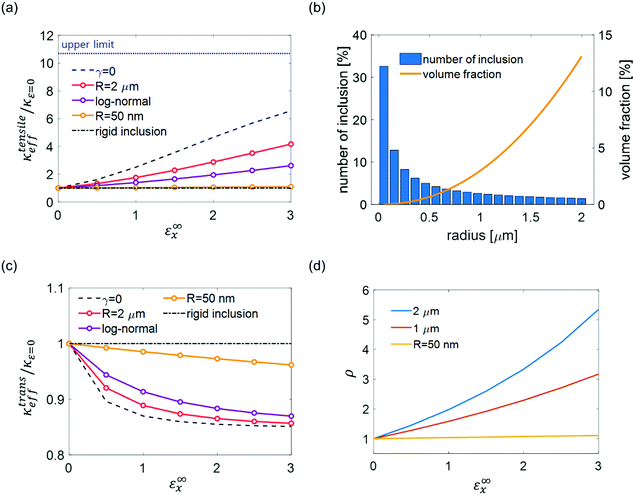Fig. 4 Tensile strain effect on effective thermal conductivities of LMCs. (a) Effective thermal conductivities in the tensile direction under the far-field strain at 20% of volume fraction. Thermal conductivities are normalized by thermal conductivity at zero strain. The upper limit in the high strain regime is represented as the blue dotted line (see SI.4, ESI† for details). (b) The assumed log-normal distribution of LM inclusions. (c) Normalized effective thermal conductivities in a transverse direction to the tensile strain under the far-field strain at 20% of volume fraction. (d) Aspect ratios of stretched inclusions of different sizes as a function of the far-field tensile strain.

According to the SEM images of the previous study, inclusion sizes have a log-normal distribution.9,38 A log-normal distribution of inclusions is assumed as shown in Fig. 4(b). The blue histogram shows the fraction of inclusions within each bin and the yellow line shows the corresponding volume fraction. When the distribution of inclusions has a histogram of N bins, the effective thermal conductivity tensor of the LMC is represented as follows,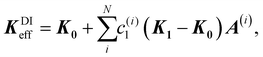(32)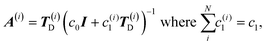(33)
where c(i)1 refers to the volume fraction and T(i)D is obtained by substituting eqn (31) to eqn (20), (21) and (24) for each inclusion size. The effective thermal conductivity with the log-normal distribution shows the values between the effective thermal conductivities obtained from R = 2 μm and R = 50 nm, showing the thermal conductivity change by almost a factor of three at 300% tensile strain (purple line in Fig. 4(a)). As the far-field strain increases, theoretical predictions converge to the upper limit, which refers to the prediction of the rule of mixture (see the SI.4, ESI). An effective thermal conductivity along the transverse direction can also be obtained from the (2, 2) or (3, 3) component of KDIeff and the result is presented in Fig. 4(c), which shows a very limited variation over the applied strain. The aspect ratio dependency of the inclusion size and the far-field strain is shown in Fig. 4(d).

We note that the contribution of the oxide layer is not accounted for in our theoretical framework. Because the oxide layer observed on the surface of LM acts like a thin and rigid shell,9,39,40 it would give rise to a decreased surface tension effect on the properties of the LMCs. Quantitative analysis on the complex interplay between the oxide layer and the liquid surface tension, and the resulting effective properties of LMCs will remain an important task for the LMC community. Also, because eqn (30) is derived for a single inclusion embedded in a matrix subjected to a far-field strain, the accuracy of our model is expected to be limited in a high strain regime when the distance between inclusions significantly reduces due to the Poisson effect.

## Conclusions

In this study, we investigated the effective thermal conductivities of LMCs using various mean-field homogenization methods based on Eshelby's solution with consideration of aspect ratios and external tensile loading. Out of four different mean-field homogenization frameworks, the differential method shows the best prediction accuracy with a relatively small error (∼10%) below 40% of the volume fraction and the double inclusion method has the best match with an acceptable error (<16%) in the entire LM volume fraction range considered in the study.

We also predicted that the effective thermal conductivity of typical LMCs, having micrometer scale inclusions with a log-normal distribution, increases by almost a factor of three along the tensile direction at 300% strain. The sensitivity of thermal conductivity to strain can be tuned by controlling the inclusion size distributions because it originates from the elastocapillary effect. Our study deepens the understanding on the thermal conductivity of LMCs and provides guidelines for designing and implementing thermal interfaces and packaging for applications. Still, the rigorous considerations of modeling the parameters of LMCs as a complex system, including the oxide skin layers between inclusions and a matrix, thermal interface resistance, and orientation distribution, will challenge future research.

## Conflicts of interest

There are no conflicts to declare.

## Acknowledgements

This work was supported by grants from National Research Foundation of Korea (2018K2A9A2A12000223) and the Swedish Foundation for International Cooperation in Research and Higher Education (KO 2017-7338). J. J. and S. R. also acknowledge the support from Basic Science Research Program (2019R1A2C4070690) through the National Research Foundation of Korea.

## Notes and references

1. J. A. Rogers, T. Someya and Y. Huang, Science, 2010, 327, 1603–1607 CrossRef CAS PubMed.
2. N. Lu and D.-H. Kim, Soft Robot., 2014, 1, 53–62 CrossRef.
3. M. Amjadi, K. U. Kyung, I. Park and M. Sitti, Adv. Funct. Mater., 2016, 26, 1678–1698 CrossRef CAS.
4. M. H. Malakooti, N. Kazem, J. Yan, C. Pan, E. J. Markvicka, K. Matyjaszewski and C. Majidi, Adv. Funct. Mater., 2019, 29, 1906098 CrossRef CAS.
5. C. Pan, E. J. Markvicka, M. H. Malakooti, J. Yan, L. Hu, K. Matyjaszewski and C. Majidi, Adv. Mater., 2019, 31, 1900663 CrossRef PubMed.
6. S. H. Jeong, S. Chen, J. Huo, E. K. Gamstedt, J. Liu, S.-L. Zhang, Z.-B. Zhang, K. Hjort and Z. Wu, Sci. Rep., 2015, 5, 18257 CrossRef CAS PubMed.
7. P. Fan, Z. Sun, Y. Wang, H. Chang, P. Zhang, S. Yao, C. Lu, W. Rao and J. Liu, RSC Adv., 2018, 8, 16232–16242 RSC.
8. R. Tutika, S. H. Zhou, R. E. Napolitano and M. D. Bartlett, Adv. Funct. Mater., 2018, 28, 1804336 CrossRef.
9. M. D. Bartlett, A. Fassler, N. Kazem, E. J. Markvicka, P. Mandal and C. Majidi, Adv. Mater., 2016, 28, 3726–3731 CrossRef CAS PubMed.
10. M. D. Bartlett, N. Kazem, M. J. Powell-Palm, X. Huang, W. Sun, J. A. Malen and C. Majidi, Proc. Natl. Acad. Sci. U. S. A., 2017, 114, 2143–2148 CrossRef CAS PubMed.
11. M. I. Ralphs, N. Kemme, P. B. Vartak, E. Joseph, S. Tipnis, S. Turnage, K. N. Solanki, R. Y. Wang and K. Rykaczewski, ACS Appl. Mater. Interfaces, 2018, 10, 2083–2092 CrossRef CAS PubMed.
12. R. Tavangar, J. M. Molina and L. Weber, Scr. Mater., 2007, 56, 357–360 CrossRef CAS.
13. F. Nosouhi Dehnavi, M. Safdari, K. Abrinia, A. Sheidaei and M. Baniassadi, Mater. Today Commun., 2020, 23, 100878 CrossRef CAS.
14. D. J. Eyre and G. W. Milton, Eur. Phys. J.: Appl. Phys., 1999, 6, 41–47 CrossRef.
15. H. L. Quang, Q.-C. He and G. Bonnet, Philos. Mag., 2011, 91, 3358–3392 CrossRef CAS.
16. S. Lee, J. Lee, B. Ryu and S. Ryu, Sci. Rep., 2018, 8, 1–11 CrossRef PubMed.
17. J. D. Eshelby, Proc. R. Soc. London, Ser. A, 1957, 241, 376–396 Search PubMed.
18. J. Qu and M. Cherkaoui, Fundamentals of micromechanics of solids, Wiley, Hoboken, 2006 Search PubMed.
19. J. Jung, S. Lee, B. Ryu and S. Ryu, Int. J. Heat Mass Transfer, 2019, 144, 118620 CrossRef.
20. I. Doghri and A. Ouaar, Int. J. Solids Struct., 2003, 40, 1681–1712 CrossRef.
21. R. McLaughlin, Int. J. Eng. Sci., 1977, 15, 237–244 CrossRef.
22. A. Norris, Mech. Mater., 1985, 4, 1–16 CrossRef.
23. M. Hori and S. Nemat-Nasser, Mech. Mater., 1993, 14, 189–206 CrossRef.
24. H. Hiroshi and T. Minoru, Int. J. Eng. Sci., 1986, 24, 1159–1172 CrossRef.
25. M. Hodes, R. Zhang, L. S. Lam, R. Wilcoxon and N. Lower, IEEE Trans. Compon., Packag., Manuf. Technol., 2013, 4, 46–56 Search PubMed.
26. J. E. Mark, Polymer data handbook, Oxford University Press, 2009 Search PubMed.
27. Z. Guo, A. Verma, X. Wu, F. Sun, A. Hickman, T. Masui, A. Kuramata, M. Higashiwaki, D. Jena and T. Luo, Appl. Phys. Lett., 2015, 106, 111909 CrossRef.
28. G. J. Weng, Mech. Mater., 2010, 42, 886–893 CrossRef.
29. S. Torquato and H. Haslach Jr, Appl. Mech. Rev., 2002, 55, B62–B63 CrossRef.
30. R. W. Style, R. Boltyanskiy, B. Allen, K. E. Jensen, H. P. Foote, J. S. Wettlaufer and E. R. Dufresne, Nat. Phys., 2015, 11, 82–87 Search PubMed.
31. F. Mancarella, R. W. Style and J. S. Wettlaufer, Proc. R. Soc. London, Ser. A, 2016, 472, 20150853 CrossRef PubMed.
32. S. Lee and S. Ryu, Eur. J. Mech. A:Solids, 2018, 72, 79–87 CrossRef.
33. G. M. Odegard, T. Gates, K. Wise, C. Park and E. Siochi, Compos. Sci. Technol., 2003, 63, 1671–1687 CrossRef CAS.
34. R. W. Style, A. Jagota, C.-Y. Hui and E. R. Dufresne, Annu. Rev. Condens. Matter Phys., 2017, 8, 99–118 CrossRef CAS.
35. R. W. Style, J. S. Wettlaufer and E. R. Dufresne, Soft Matter, 2015, 11, 672–679 RSC.
36. S. H. Jeong, A. Hagman, K. Hjort, M. Jobs, J. Sundqvist and Z. Wu, Lab Chip, 2012, 12, 4657–4664 RSC.
37. V. Kocourek, C. Karcher, M. Conrath and D. Schulze, Phys. Rev. E: Stat., Nonlinear, Soft Matter Phys., 2006, 74, 026303 CrossRef CAS PubMed.
38. Z. J. Farrell and C. Tabor, Langmuir, 2018, 34, 234–240 CrossRef CAS PubMed.
39. H. Liang, Y. Zeng, K. Zuo, X. Xia, D. Yao and J. Yin, Ceram. Int., 2017, 43, 5517–5523 CrossRef CAS.
40. E. Ricci, E. Arato, A. Passerone and P. Costa, Adv. Colloid Interface Sci., 2005, 117, 15–32 CrossRef CAS PubMed.

### Footnote

Electronic supplementary information (ESI) available. See DOI: 10.1039/d0sm00279h
 This journal is © The Royal Society of Chemistry 2020